# Algebra Intermediate - Factoring Polynomials [Step 2: 4 Terms]

This Math quiz is called 'Algebra Intermediate - Factoring Polynomials [Step 2: 4 Terms]' and it has been written by teachers to help you if you are studying the subject at middle school. Playing educational quizzes is a fabulous way to learn if you are in the 6th, 7th or 8th grade - aged 11 to 14.

It costs only \$12.50 per month to play this quiz and over 3,500 others that help you with your school work. You can subscribe on the page at Join Us

This quiz is going to deal with working with Step 2 in factoring polynomials. If you skipped Step 1, now is the time to find that quiz, read the introduction and do the ten problems. You will need to understand that step before moving forward to Step 2.

As you should recall by now, the word “factor” or “factoring” tells us that we will be multiplying. When factoring polynomials, there are a number of steps. Step 1 was to find the greatest common factor or, in other words, when you have 2 or more numbers, which highest number do each of those numbers have that when multiplied by another number will equal that number.

For example, let’s look at the numbers 6 and 12. What would be their greatest common factor? Well, 1 x 6 = 6 and 2 x 6 = 12 so this shows us that the common greatest factor is the number “6”. Once you determine the greatest common factor and have found a solution, it is time to move on to Step 2.

STEP 2: Find the Number of Terms

As you have previously learned, a number and/or a variable is referred to as a “term” and one number or variable, by itself, is known as a monomial. Two monomials in an equation (also called a problem or an expression) are known as a binomial. Three terms in an equation are known as trinomials and four or more terms in an equation are known as a polynomial. However, 2 terms and 3 terms in a problem can also be referred to as polynomials. So, in Step 2 of factoring polynomials you will need to determine how many terms are involved. The number of terms will directly affect the “grouping” of terms. In other words, terms that are the same need to be grouped together. For this quiz we will focus on four terms. Now, let’s look at the problem below that has four terms.

Go straight to Quiz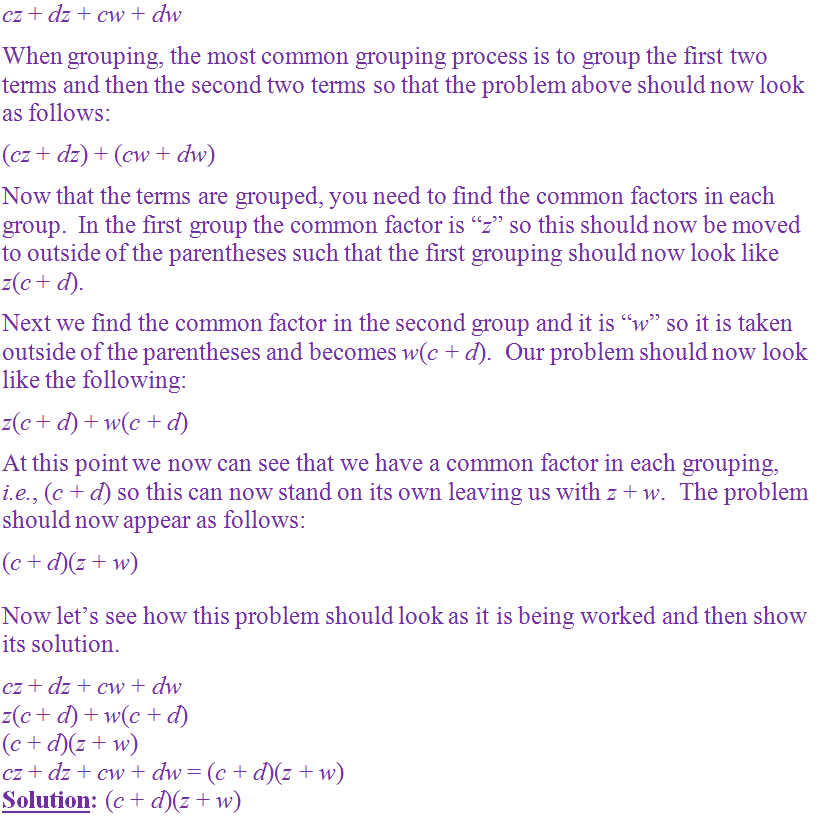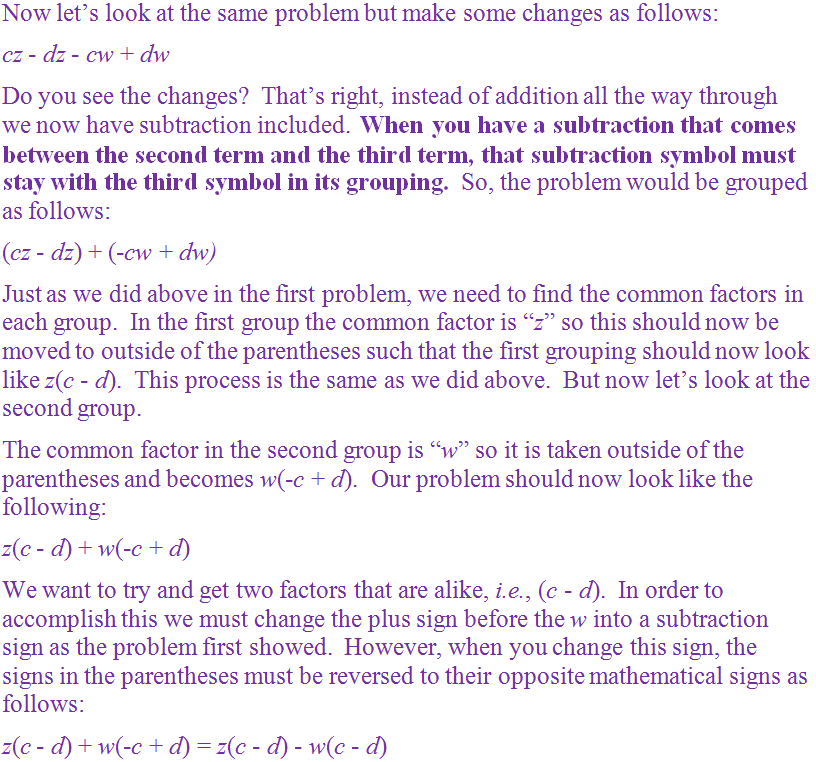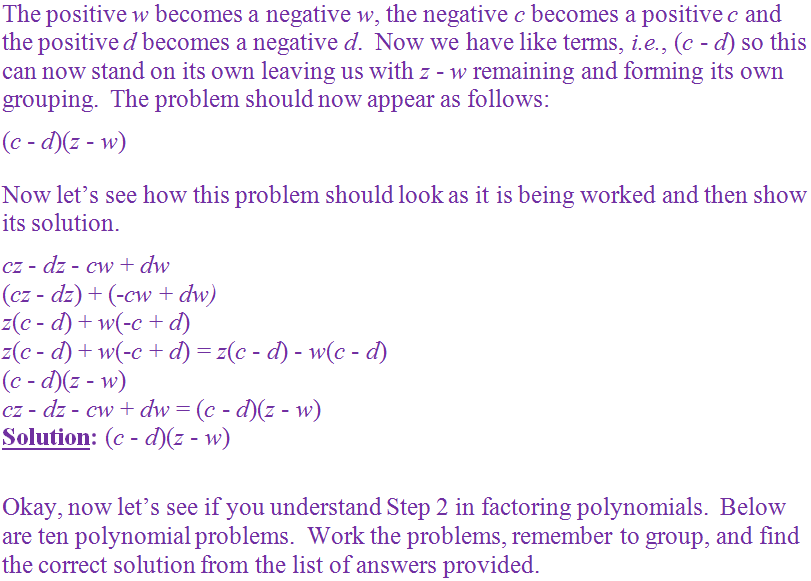1.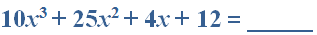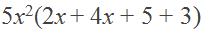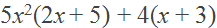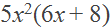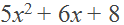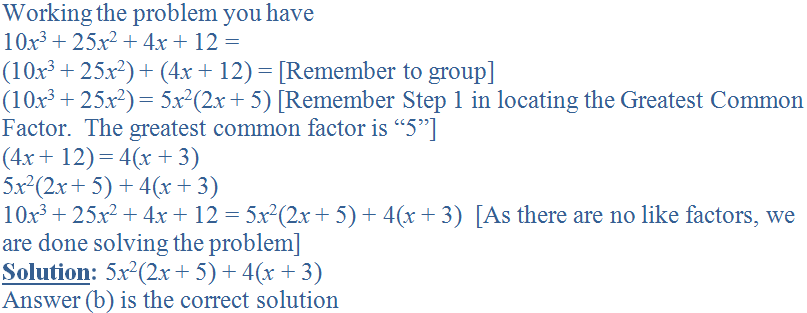2.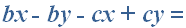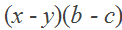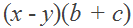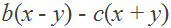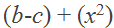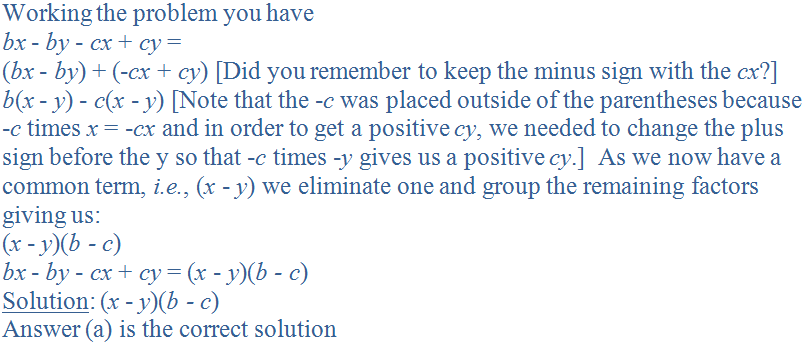3.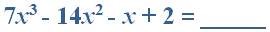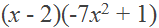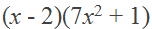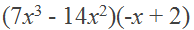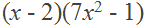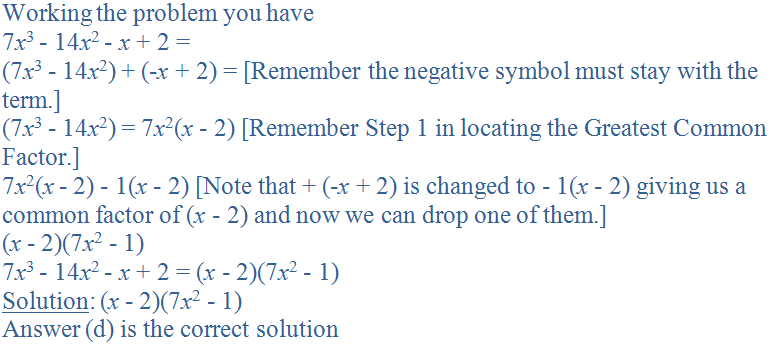4.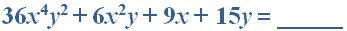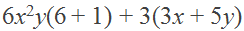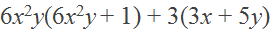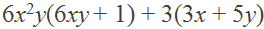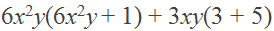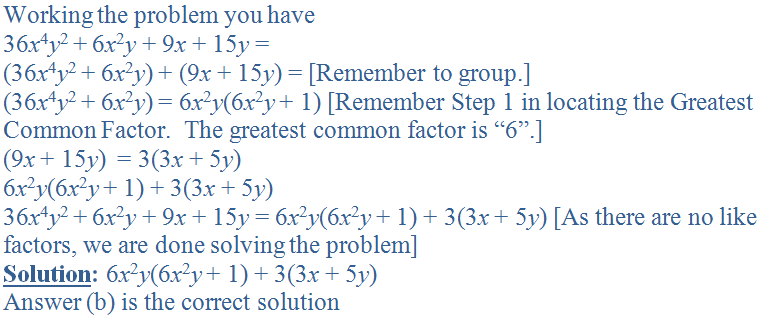5.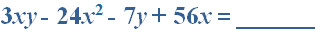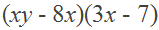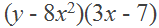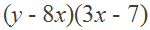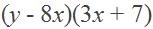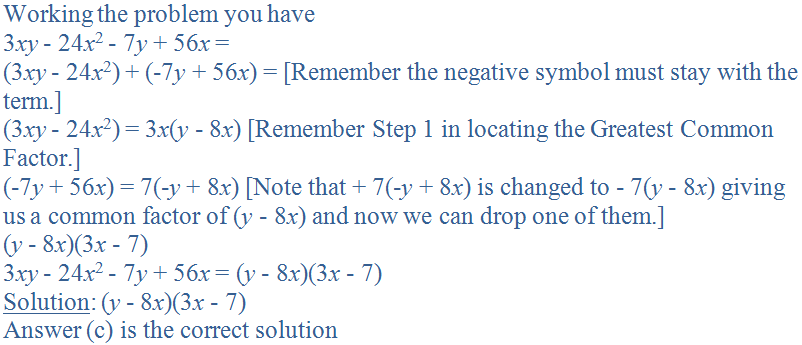6.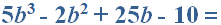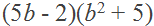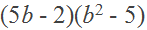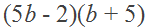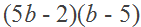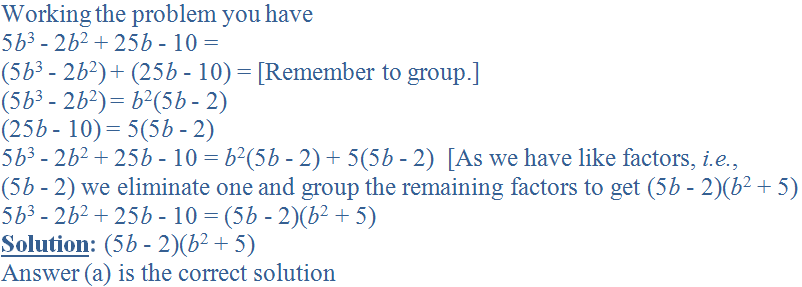7.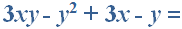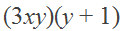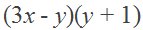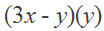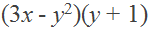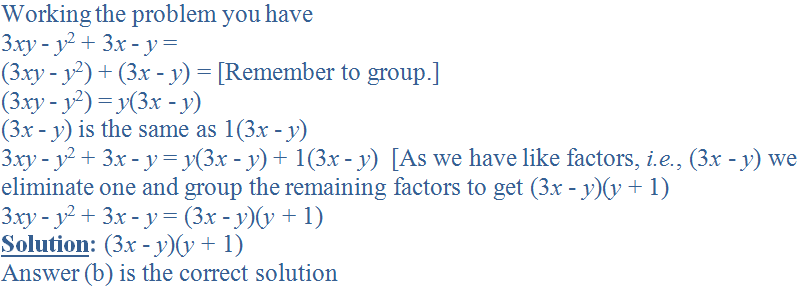8.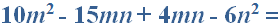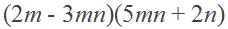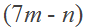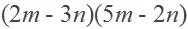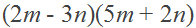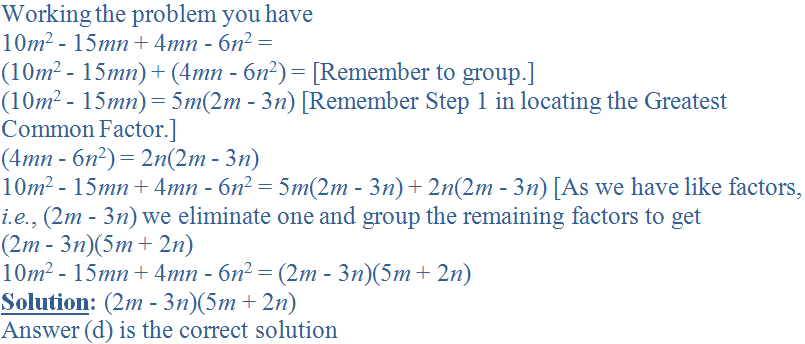9.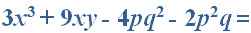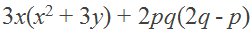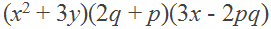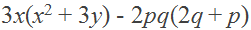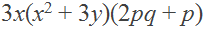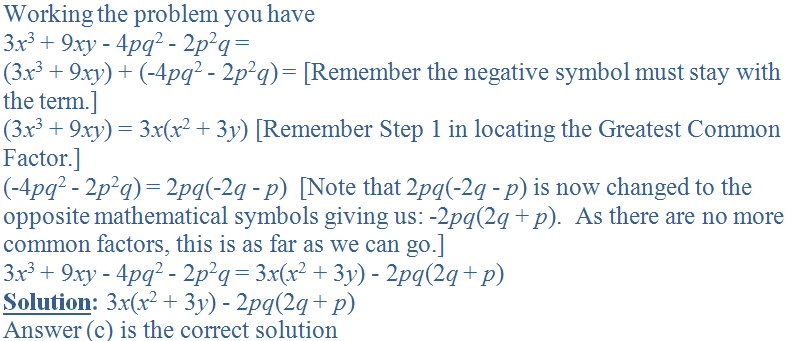10.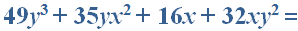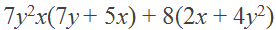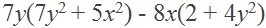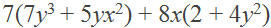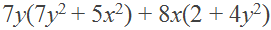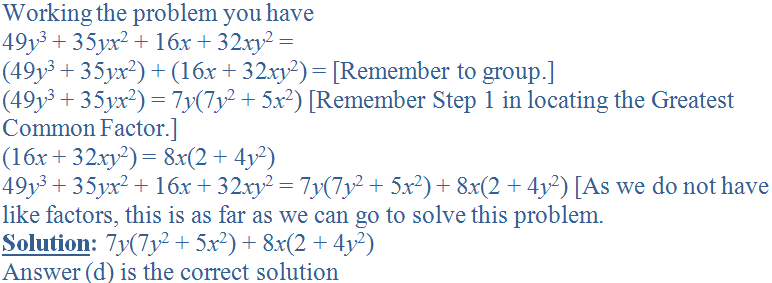Author:  Christine G. Broome